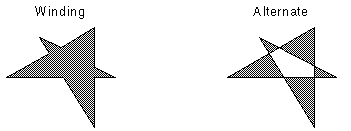←Select platform
In This Topic ▼

Summary

Syntax
C#
VB
C++
Java
````public void AddPolygonToRegion( `
`   RasterRegionXForm xform, `
`   IList<LeadPoint> points, `
`   LeadFillMode fillMode, `
`   RasterRegionCombineMode combineMode `
`) ````
````Public Overloads Sub AddPolygonToRegion( _ `
`   ByVal xform As RasterRegionXForm, _ `
`   ByVal points As IList(Of LeadPoint), _ `
`   ByVal fillMode As LeadFillMode, _ `
`   ByVal combineMode As RasterRegionCombineMode _ `
`)  ````
````public void addPolygonToRegion( `
`  RasterRegionXForm xform,  `
`  List<LeadPoint> points,  `
`  Path.FillType fillType,  `
`  RasterRegionCombineMode combineMode `
`) ````
````public: `
`void AddPolygonToRegion(  `
`   RasterRegionXForm^ xform, `
`   IList<LeadPoint>^ points, `
`   LeadFillMode fillMode, `
`   RasterRegionCombineMode combineMode `
`)  ````

#### Parameters

xform
RasterRegionXForm object that LEADTOOLS uses to translate between display coordinates and image coordinates. If you specify null in this parameter, the scalar fields default to 1, the offsets default to 0, and the view perspective defaults to the image view perspective.

points
A collection of LeadPoint objects. The points in the collection must be in the order in which the vertices of the polygon are connected. To create the line that closes the polygon, the last point in the collection is connected to the first point of the collection.

fillMode
Indicates how to handle complex crossing lines.

Value Meaning
LeadPoint.Winding All pixels that are inside the resulting exterior lines are in the region.
LeadFillMode.Alternate The region includes the area between odd-numbered and even-numbered polygon sides on each scan line.combineMode
The action to take regarding the existing image region, if one is defined.

Remarks

To update an existing region, you specify how the new region is to be combined with the existing one using the combineMode parameter. For more information, refer to RasterRegionCombineMode.

Example

This example will load an image, add a polygon region to it, and then run the InvertCommand to show the affected area before saving the image back to disk.

C#
VB
````using Leadtools; `
`using Leadtools.Codecs; `
`using Leadtools.ImageProcessing; `
`using Leadtools.ImageProcessing.Core; `
`using Leadtools.ImageProcessing.Color; `
`using Leadtools.Dicom; `
`using Leadtools.Drawing; `
`using Leadtools.Controls; `
`using LeadtoolsExamples.Common; `
`using Leadtools.Svg; `
` `
`public void AddPolygonToRegionExample() `
`{ `
`   RasterCodecs codecs = new RasterCodecs(); `
` `
`   string srcFileName = Path.Combine(ImagesPath.Path, "Image1.cmp"); `
`   string destFileName = Path.Combine(ImagesPath.Path, "Image1_AddPolygonToRegion.bmp"); `
` `
`   // Load the image `
`   RasterImage image = codecs.Load(srcFileName); `
` `
`   // Add a polygon region `
` `
`   RasterRegionXForm xform = new RasterRegionXForm(); `
`   xform.ViewPerspective = RasterViewPerspective.TopLeft; `
`   xform.XOffset = 0; `
`   xform.YOffset = 0; `
`   xform.XScalarDenominator = 1; `
`   xform.XScalarNumerator = 1; `
`   xform.YScalarDenominator = 1; `
`   xform.YScalarNumerator = 1; `
` `
`   int x1 = image.ImageWidth / 4; `
`   int y1 = image.ImageHeight / 4; `
`   int x2 = image.ImageWidth / 3; `
`   int y2 = image.ImageHeight / 3; `
` `
`   LeadPoint[] pts = `
`   { `
`      new LeadPoint(x1, y1), `
`      new LeadPoint(x2, y1), `
`      new LeadPoint(x1, y2), `
`      new LeadPoint(x2, y2) `
`   }; `
` `
`   image.AddPolygonToRegion(xform, pts, LeadFillMode.Winding, RasterRegionCombineMode.Set); `
` `
`   // Draw something on the image `
`   InvertCommand command = new InvertCommand(); `
`   command.Run(image); `
` `
`   // Save the image `
`   codecs.Save(image, destFileName, RasterImageFormat.Bmp, 24); `
` `
`   image.Dispose(); `
`   codecs.Dispose(); `
`} ````
````Imports Leadtools `
`Imports Leadtools.Codecs `
`Imports Leadtools.ImageProcessing `
`Imports Leadtools.ImageProcessing.Core `
`Imports Leadtools.ImageProcessing.Color `
`Imports Leadtools.Controls `
`Imports Leadtools.Dicom `
`Imports Leadtools.Drawing `
`Imports Leadtools.Svg `
` `
`Public Sub AddPolygonToRegionExample() `
`   Dim codecs As RasterCodecs = New RasterCodecs() `
` `
`   Dim srcFileName As String = Path.Combine(LEAD_VARS.ImagesDir, "Image1.cmp") `
`   Dim destFileName As String = Path.Combine(LEAD_VARS.ImagesDir, "Image1_AddPolygonToRegion.bmp") `
` `
`   ' Load the image `
`   Dim image As RasterImage = codecs.Load(srcFileName) `
` `
`   ' Add a polygon region `
` `
`   Dim xform As RasterRegionXForm = New RasterRegionXForm() `
`   xform.ViewPerspective = RasterViewPerspective.TopLeft `
`   xform.XOffset = 0 `
`   xform.YOffset = 0 `
`   xform.XScalarDenominator = 1 `
`   xform.XScalarNumerator = 1 `
`   xform.YScalarDenominator = 1 `
`   xform.YScalarNumerator = 1 `
` `
`   Dim x1 As Integer = image.ImageWidth \ 4 `
`   Dim y1 As Integer = image.ImageHeight \ 4 `
`   Dim x2 As Integer = image.ImageWidth \ 3 `
`   Dim y2 As Integer = image.ImageHeight \ 3 `
` `
`   Dim pts As LeadPoint() = {New LeadPoint(x1, y1), New LeadPoint(x2, y1), New LeadPoint(x1, y2), New LeadPoint(x2, y2)} `
` `
`   image.AddPolygonToRegion(xform, pts, LeadFillMode.Winding, RasterRegionCombineMode.Set) `
` `
`   ' Draw something on the image `
`   Dim command As InvertCommand = New InvertCommand() `
`   command.Run(image) `
` `
`   ' Save the image `
`   codecs.Save(image, destFileName, RasterImageFormat.Bmp, 24) `
` `
`   image.Dispose() `
`   codecs.Dispose() `
`End Sub `
` `
`Public NotInheritable Class LEAD_VARS `
`   Public Const ImagesDir As String = "C:\Users\Public\Documents\LEADTOOLS Images" `
`End Class ````

Requirements